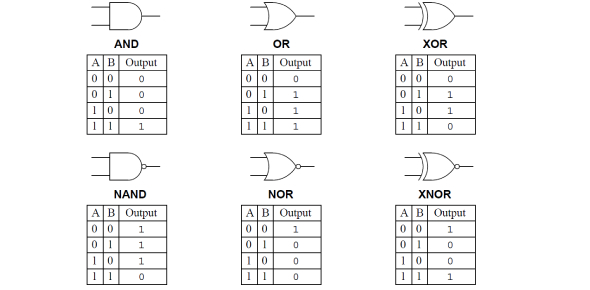# Know Everything About Logic Gate! Electronic Quiz

Approved & Edited by ProProfs Editorial Team
At ProProfs Quizzes, our dedicated in-house team of experts takes pride in their work. With a sharp eye for detail, they meticulously review each quiz. This ensures that every quiz, taken by over 100 million users, meets our standards of accuracy, clarity, and engagement.
| Written by Alex_yeo
A
Alex_yeo
Community Contributor
Quizzes Created: 1 | Total Attempts: 10,573
Questions: 5 | Attempts: 10,607SettingsIn the practice of electronics, a logic gate is a device which carries out a logical operation on a certain number of binary inputs and produces just one binary output. In the following quiz, we aim to put your knowledge on these logic gates to the test, offering up tables, diagrams and more to gauge the level of understanding you have on the topic. Think you can get them all right? Let’s find out!

• 1.

### State the name of the logic gate shown.

• A.

NAND Gate

• B.

NOR Gate

• C.

NOT Gate

• D.

OR Gate

• E.

AND Gate

D. OR Gate
Explanation
The given logic gate is an OR gate. An OR gate is a digital logic gate that outputs true (1) if at least one of its inputs is true. In this case, the gate is represented by a symbol that resembles the letter "O", which is commonly used to represent an OR gate.

Rate this question:

• 2.

### Which logic gate does this truth table belong to?

• A.

AND Gate

• B.

NAND Gate

• C.

OR Gate

• D.

NOR Gate

• E.

NOT Gate

• F.

None of the above

B. NAND Gate
Explanation
The given truth table represents a NAND gate. A NAND gate produces a low output only when both of its inputs are high. In all other cases, it produces a high output. By looking at the truth table, we can see that the output is low (0) only when both inputs are high (1,1). In all other cases, the output is high (1). Therefore, the given truth table matches the behavior of a NAND gate.

Rate this question:

• 3.

### Identify the truth table for a NOR gate.

• A.
• B.
• C.
• D.
• E.

None of the above

A.
• 4.

### Identify the symbol used to represent the NOT gate.

• A.
• B.
• C.
• D.
• E.

None of the above

E. None of the above
Explanation
The question asks for the symbol used to represent the NOT gate. However, the answer states "None of the above," indicating that none of the options provided in the question are correct. Since the question does not provide any options, it is not possible to determine the correct symbol for the NOT gate.

Rate this question:

• 5.

### Convert the following word problem into a logic working.  The light bulb(L) is ON if   switch A (A) is OFF and switch B (B) is ON   and either switch B (B) is OFF or switch (C) is ON

Related TopicsBack to top
×

Wait!
Here's an interesting quiz for you.